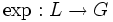# Lazard correspondence establishes a correspondence between powering-invariant characteristic subgroups and powering-invariant characteristic subrings

Suppose$G$ is a Lazard Lie group,$L$ is its Lazard Lie ring, and$\exp:L \to G$ and$\log:G \to L$ are the exponential and logarithm maps respectively (they are both bijections and are inverses of each other). Note that we may wish to think of$G$ and$L$ as having the same underlying set and treat the bijections as being the identity map on the underlying set; however, for conceptual convenience, we are using separate symbols for the group and Lie ring and explicit names for the bijections.
powering-invariant characteristic subgroups of$G$$\leftrightarrow$ powering-invariant characteristic subrings of$L$